You may also likeInclusion Exclusion

How many integers between 1 and 1200 are NOT multiples of any of the numbers 2, 3 or 5?Rain

How many dry days did Aisha have on her holiday?

Clubs

Age 11 to 14 ShortChallenge Level

Using words and trial
Think about the people in both clubs. Suppose that there are 10 people in the maths club who are in both clubs. Then those 10 people are also in the science club. And there can't be any other people in the science club who are in both clubs, because if there were, then they would be in the maths club - but we have already counted the 10 people in the maths club who are in both clubs!

So if there are 10 people in the maths club who are in both clubs, then there are also 10 people in the science club who are in both clubs - and they are the same people.

If there are 10 people who are in both clubs, then there are 15$-$10 = 5 people in just the maths club, and 12$-$10 = 2 people in just the science club. That is a total of 7 people in only one club, which is 6 less than 13.

10$-$6 = 4, so what if there are 4 people in both clubs? Then there are 11 people in just the maths club, and 8 people just in the science club. That is a total of 19 people in only one club, which is 6 more than 13.

So we could try half-way between 4 and 10 - what if there are 7 people in both clubs? Then there are 8 people in just the maths club, and 5 people in just the science club. That is a total of 13 people in only one club - perfect.

Using trial on a venn diagram
In this first Venn diagram, the 15 students in the maths club and the 12 students in the science club are all shown as dots, and all shown as being in only one club.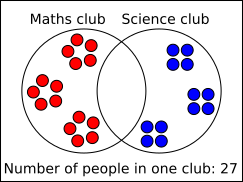Putting a student into the overlap is the same as moving one student from each side, since the student in the overlap is in both clubs - there are still 15 students in the maths club and 12 students in the science club.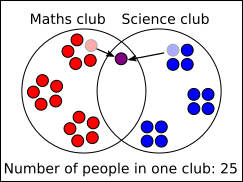So for every student put into the overlap, the number of students in just one club goes down by 2. We can keep going until the number of people in one club is 13.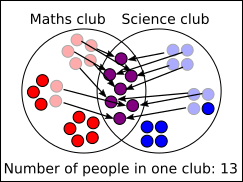There are 7 people in both clubs.

Using algebra on a Venn diagram
There are 15 people in the maths club and 12 in the science club, so 15 people in the red circle and 12 in the blue circle.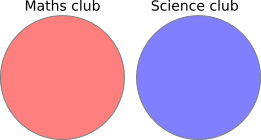The two circles can be overlapped to form a Venn diagram, where there are 13 people in the area shaded purple, because there are 13 people in just one club. We are asked for the number of people in the green area - in both clubs.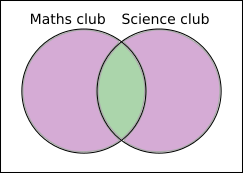The total shaded area is 13 + green area.

Adding the red circle to the blue circle gives the same number, except that the overlap is counted twice, giving 13 + 2$\times$green area instead of 13 + green area.

So 15 + 12 = 13 + 2$\times$green area $\Rightarrow$ 14 = 2$\times$green area, so there are 7 students in both clubs.

Using a two-way table
This two-way table is filled in in terms of $x$, the number of students in both clubs.
Science club
Maths club   Yes No Total
Yes $x$ $15-x$ $15$
No $12-x$
Total $12$
We know that $15-x$ and $12-x$ add up to $13$, so $15-x+12-x=13$.

Collecting like terms, $27-2x=13$, so $27=2x+13$, so $2x = 14$ so $x=7$.

Can you see where each of these terms would go on the Venn diagram?

You can find more short problems, arranged by curriculum topic, in our short problems collection.